# TextCNN文本分类（keras实现）

1、读取数据集

2、将文字转换成数字特征

3、将每条文本转换为数字列表

4、将每条文本设置为相同长度

5、将每个词编码转换为词向量

6、Keras文本预处理代码实现

1、基础版CNN（模仿LeNet-5）

2、简单版TextCNN

3、使用Word2Vec词向量的TextCNN

1、环境配置

2、绘制模型图

## 一、论文笔记

1、Yoon Kim在2014年 “Convolutional Neural Networks for Sentence Classification” 论文中提出TextCNN（利用卷积神经网络对文本进行分类的算法）（该论文翻译）。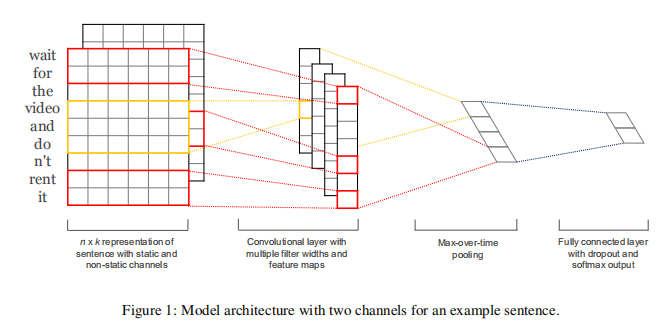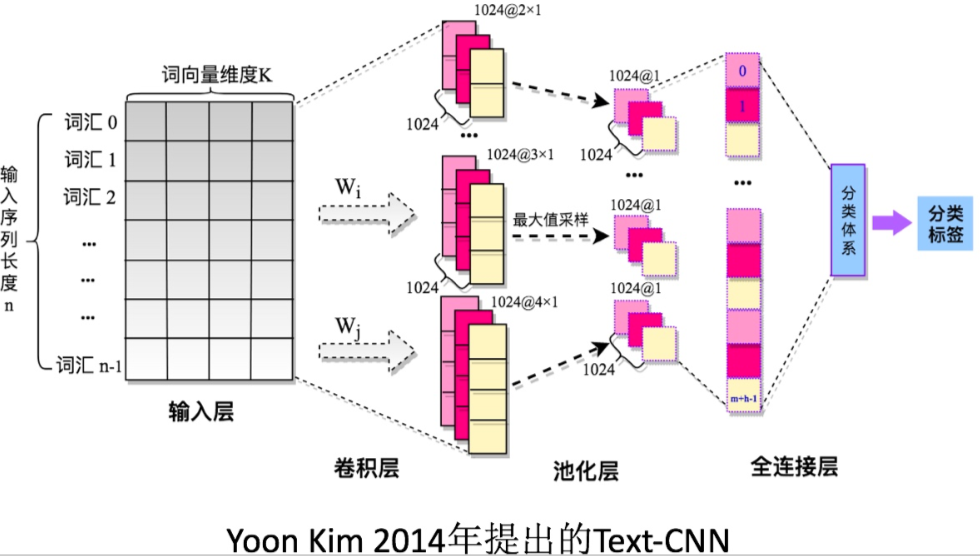（1）嵌入层（Embedding Layer）

（2）卷积层（Convolution Laye）

（3）池化层（Pooling Layer）

（4）全连接层（Fully connected layer）

（5）TextCNN的小变种

（6）参数与超参数

• sequence_length （Q: 对于CNN, 输入与输出都是固定的，可每个句子长短不一, 怎么处理? A: 需要做定长处理, 比如定为n, 超过的截断, 不足的补0. 注意补充的0对后面的结果没有影响，因为后面的max-pooling只会输出最大值，补零的项会被过滤掉）
• num_classes （多分类, 分为几类）
• vocabulary_size （语料库的词典大小, 记为|D|）
• embedding_size （将词向量的维度, 由原始的 |D| 降维到 embedding_size）
• filter_size_arr （多个不同size的filter）

2、2015年“A Sensitivity Analysis of (and Practitioners' Guide to) Convolutional Neural Networks for Sentence Classification”论文详细地阐述了关于TextCNN模型的调参心得。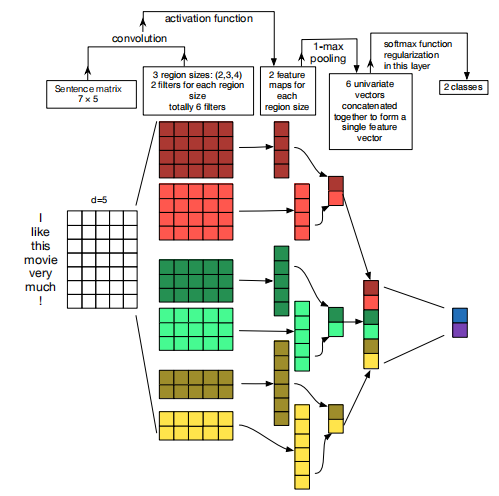（1）TextCNN详细过程：

• Embedding：第一层是图中最左边的7乘5的句子矩阵，每行是词向量，维度=5，这个可以类比为图像中的原始像素点。
• Convolution：然后经过 kernel_sizes=(2,3,4) 的一维卷积层，每个kernel_size 有两个输出 channel。
• MaxPolling：第三层是一个1-max pooling层，这样不同长度句子经过pooling层之后都能变成定长的表示。
• FullConnection and Softmax：最后接一层全连接的 softmax 层，输出每个类别的概率。

（2）论文调参结论：

• 使用预训练的word2vec 、 GloVe初始化效果会更好。一般不直接使用One-hot。
• 卷积核的大小影响较大，一般取1~10，对于句子较长的文本，则应选择大一些。
• 卷积核的数量也有较大的影响，一般取100~600 ，同时一般使用Dropout（0~0.5）。
• 激活函数一般选用ReLU 和 tanh。
• 池化使用1-max pooling。
• 随着feature map数量增加，性能减少时，试着尝试大于0.5的Dropout。
• 评估模型性能时，记得使用交叉验证。

## 二、Keras文本预处理

### 5、将每个词编码转换为词向量

Embedding层基于上文所得的词编码，对每个词进行one-hot编码，每个词都会是一个vocabulary_size维的向量；然后通过神经网络的训练迭代更新得到一个合适的权重矩阵（具体实现过程可以参考skip-gram模型），行大小为vocabulary_size，列大小为词向量的维度，将本来以one-hot编码的词向量映射到低维空间，得到低维词向量。需要声明一点的是Embedding层是作为模型的第一层，在训练模型的同时，得到该语料库的词向量。当然，也可以使用已经预训练好的词向量表示现有语料库中的词。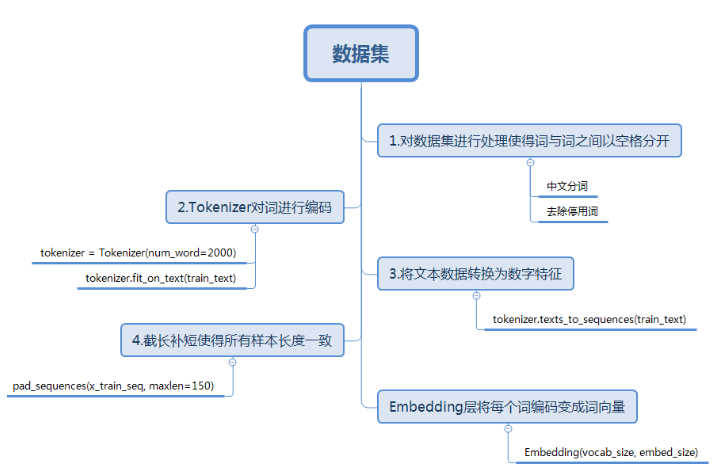### 6、Keras文本预处理代码实现

from sklearn.model_selection import train_test_split
import pandas as pd
import jieba
from keras.preprocessing.text import Tokenizer

if __name__=='__main__':
dataset = pd.read_csv('sentiment_analysis/data_train.csv', sep='\t',names=['ID', 'type', 'review', 'label']).astype(str)
cw = lambda x: list(jieba.cut(x))
dataset['words'] = dataset['review'].apply(cw)
tokenizer=Tokenizer()  #创建一个Tokenizer对象
#fit_on_texts函数可以将输入的文本中的每个词编号，编号是根据词频的，词频越大，编号越小
tokenizer.fit_on_texts(dataset['words'])
vocab=tokenizer.word_index #得到每个词的编号
x_train, x_test, y_train, y_test = train_test_split(dataset['words'], dataset['label'], test_size=0.1)
# 将每个样本中的每个词转换为数字列表，使用每个词的编号进行编号
x_train_word_ids=tokenizer.texts_to_sequences(x_train)
x_test_word_ids = tokenizer.texts_to_sequences(x_test)
#序列模式
# 每条样本长度不唯一，将每条样本的长度设置一个固定值


## 三、基于keras的TextCNN模型的构建、训练与测试

### 1、基础版CNN（模仿LeNet-5）

LeNet-5是卷积神经网络的作者Yann LeCun用于MNIST识别任务提出的模型。模型很简单，就是卷积池化层的堆叠，最后加上几层全连接层。将其运用在文本分类任务中。

#构建CNN分类模型(LeNet-5)
#模型结构：嵌入-卷积池化*2-dropout-BN-全连接-dropout-全连接
model = Sequential()
model.add(Embedding(len(vocab) + 1, 300, input_length=50)) #使用Embeeding层将每个词编码转换为词向量
one_hot_labels = keras.utils.to_categorical(y_train, num_classes=3)  # 将标签转换为one-hot编码
y_predict = list(map(str, y_predict))
print('准确率', metrics.accuracy_score(y_test, y_predict))
print('平均f1-score:', metrics.f1_score(y_test, y_predict, average='weighted'))

### 2、简单版TextCNN

#构建TextCNN模型
#模型结构：词嵌入-卷积池化*3-拼接-全连接-dropout-全连接
main_input = Input(shape=(50,), dtype='float64')
# 词嵌入（使用预训练的词向量）
embedder = Embedding(len(vocab) + 1, 300, input_length=50, trainable=False)
embed = embedder(main_input)
# 词窗大小分别为3,4,5
cnn1 = Conv1D(256, 3, padding='same', strides=1, activation='relu')(embed)
cnn1 = MaxPooling1D(pool_size=48)(cnn1)
cnn2 = Conv1D(256, 4, padding='same', strides=1, activation='relu')(embed)
cnn2 = MaxPooling1D(pool_size=47)(cnn2)
cnn3 = Conv1D(256, 5, padding='same', strides=1, activation='relu')(embed)
cnn3 = MaxPooling1D(pool_size=46)(cnn3)
# 合并三个模型的输出向量
cnn = concatenate([cnn1, cnn2, cnn3], axis=-1)
flat = Flatten()(cnn)
drop = Dropout(0.2)(flat)
main_output = Dense(3, activation='softmax')(drop)
model = Model(inputs=main_input, outputs=main_output)

one_hot_labels = keras.utils.to_categorical(y_train, num_classes=3)  # 将标签转换为one-hot编码
#y_test_onehot = keras.utils.to_categorical(y_test, num_classes=3)  # 将标签转换为one-hot编码
result_labels = np.argmax(result, axis=1)  # 获得最大概率对应的标签
y_predict = list(map(str, result_labels))
print('准确率', metrics.accuracy_score(y_test, y_predict))
print('平均f1-score:', metrics.f1_score(y_test, y_predict, average='weighted'))

### 3、使用Word2Vec词向量的TextCNN

w2v_model=Word2Vec.load('sentiment_analysis/w2v_model.pkl')
# 预训练的词向量中没有出现的词用0向量表示
embedding_matrix = np.zeros((len(vocab) + 1, 300))
for word, i in vocab.items():
try:
embedding_vector = w2v_model[str(word)]
embedding_matrix[i] = embedding_vector
except KeyError:
continue

#构建TextCNN模型
# 模型结构：词嵌入-卷积池化*3-拼接-全连接-dropout-全连接
main_input = Input(shape=(50,), dtype='float64')
# 词嵌入（使用预训练的词向量）
embedder = Embedding(len(vocab) + 1, 300, input_length=50, weights=[embedding_matrix], trainable=False)
#embedder = Embedding(len(vocab) + 1, 300, input_length=50, trainable=False)
embed = embedder(main_input)
# 词窗大小分别为3,4,5
cnn1 = Conv1D(256, 3, padding='same', strides=1, activation='relu')(embed)
cnn1 = MaxPooling1D(pool_size=38)(cnn1)
cnn2 = Conv1D(256, 4, padding='same', strides=1, activation='relu')(embed)
cnn2 = MaxPooling1D(pool_size=37)(cnn2)
cnn3 = Conv1D(256, 5, padding='same', strides=1, activation='relu')(embed)
cnn3 = MaxPooling1D(pool_size=36)(cnn3)
# 合并三个模型的输出向量
cnn = concatenate([cnn1, cnn2, cnn3], axis=-1)
flat = Flatten()(cnn)
drop = Dropout(0.2)(flat)
main_output = Dense(3, activation='softmax')(drop)
model = Model(inputs=main_input, outputs=main_output)

one_hot_labels = keras.utils.to_categorical(y_train, num_classes=3)  # 将标签转换为one-hot编码
#y_test_onehot = keras.utils.to_categorical(y_test, num_classes=3)  # 将标签转换为one-hot编码
result_labels = np.argmax(result, axis=1)  # 获得最大概率对应的标签
y_predict = list(map(str, result_labels))
print('准确率', metrics.accuracy_score(y_test, y_predict))
print('平均f1-score:', metrics.f1_score(y_test, y_predict, average='weighted'))

## 四、绘制TextCNN模型结构图

### 1、环境配置

（1）安装graphviz模块

（2）安装pydot模块

（3）在运行程序中加入下面两行代码

import os
os.environ["PATH"] += os.pathsep + 'C:/Program Files (x86)/Graphviz2.38/bin/'

### 2、绘制模型图

from keras.utils import plot_model
#生成一个模型图，第一个参数为模型，第二个参数为要生成图片的路径及文件名，还可以指定两个参数：
#show_shapes:指定是否显示输出数据的形状，默认为False
#show_layer_names:指定是否显示层名称，默认为True
plot_model(model,to_file='sentiment_analysis/model.png',show_shapes=True,show_layer_names=False)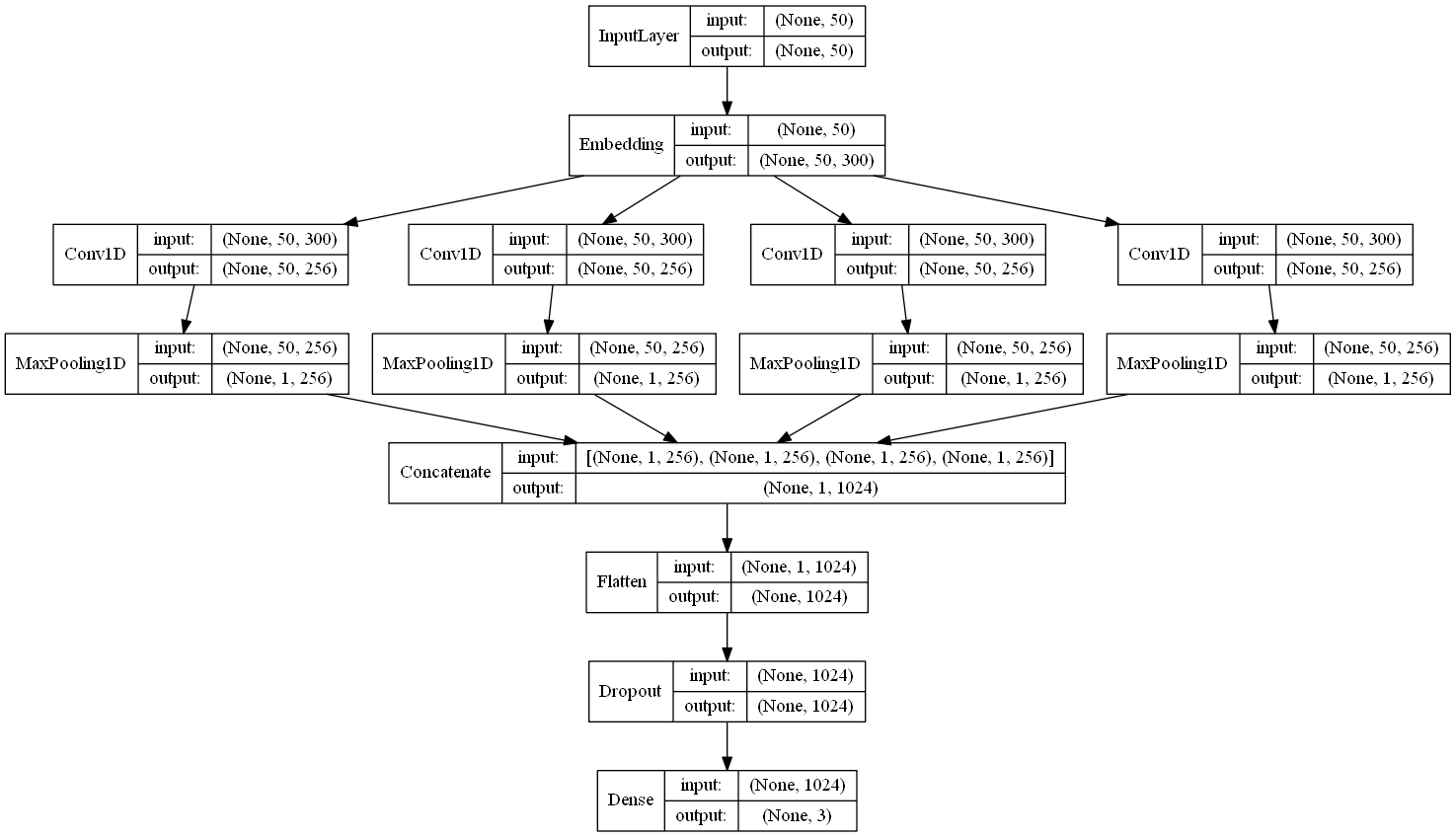## 五、keras模型的保存与加载

from keras.models import load_model

#模型的保存
model.save('model.h5')

#模型的加载
model=load_model('model.h5')

（1）Keras之文本分类实现

（2）使用Keras进行深度学习

（3）NLP论文

（4）卷积神经网络（CNN）在句子建模上的应用

（6）深度学习在文本分类中的应用

（7）深度学习与文本分类总结第一篇--常用模型总结

12-22701307-241万+
03-191877
03-072805
04-253184
07-318761
02-09
03-152000
02-271321
06-271万+
09-081529
04-201万+
01-25892
01-10718
09-205059
09-111689
01-092万+